>### Master Math with 1:1 Online Classes. Learn from Experts!# Integers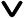• Integers Definition
• Symbol for Integers
• Types of Integers
• Zero
• Positive Integers
• Negative Integers
• Integers on Number line
• Operations on integers
• Subtraction of Integers
• Multiplication of Integers
• Division of Integers
• Properties of Integers
• Closure Property
• Commutative Property
• Associative Property
• Distributive Property
• Multiplicative Inverse Property
• Identity Property
• Solved Examples
• Practice Questions

Integers are a fundamental concept in mathematics, representing whole numbers and their negatives. In simple terms, they are numbers without any fractional or decimal parts. Integers include positive numbers, negative numbers, and zero. They are essential in various mathematical operations and real-life applications.

## Integers Definition

Integers are derived from the Latin word "integer," meaning "whole" or "intact". So, Integers include all whole numbers including negative numbers that do not have any fractional or decimal components. The set of integers is infinite and spans in both positive and negative directions.### Symbol for Integers

The symbol used to represent the set of integers is "ℤ." This symbol comes from the German word "Zahlen," which means "numbers." In mathematical notation, you may see this symbol in various contexts to indicate that the numbers under discussion are integers.## Types of Integers

Integers can be categorized into three main types: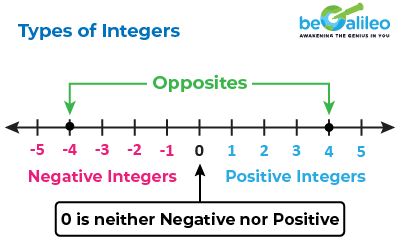### Zero

Zero is a special integer that represents the neutral point on the number line. It is neither positive nor negative and serves as the additive identity, meaning that any integer added to zero remains unchanged. In mathematical notation, it is denoted by "0."

### Positive Integers

Positive integers are numbers greater than zero. They are represented with or without a positive sign and form an infinite sequence that extends to the right on the number line. In mathematical notation, positive integers are denoted as (+1, +2, +3, ...).

### Negative Integers

Negative integers are numbers less than zero. They are represented with a negative sign and form an infinite sequence that extends to the left on the number line. In mathematical notation, negative integers are denoted as (-1, -2, -3, ...).

## Integers on the Number Line

Visualizing integers on the number line is a useful way to understand their properties and relationships. The number line extends infinitely in both positive and negative directions, with zero at its center. Positive integers lie to the right, negative integers to the left, and zero at the center.

Integers are numbers with a direction, one direction is positive, and the other is negative. Hence, integer is called a directed number that is preceded by a plus or minus sign.

Two integers are opposites if they are at the same distance from 0 in either the positive or negative direction on the number line.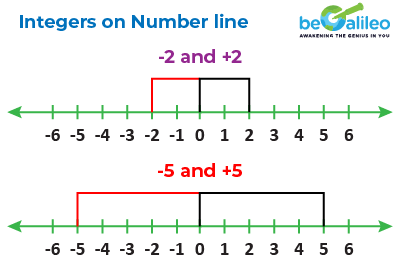### Absolute Value of Integer

On the number line, the actual distance of the integer from zero is called the absolute value.

Absolute value of a number is represented by two vertical lines.

|-7| = 7 and |15| = 15

• The absolute value of any integer is the number itself.
• The absolute value is always positive.

### Comparing Integers• Positive numbers are always greater than zero and negative numbers are always smaller than zero.
• The number on the right side is always greater than the number on the left side.

For example:

• -3 < 3
• -3 < 0
• -3 < -2
• -5 < -3

## Operations on integers

There are 4 basic arithmetic operations that can be performed with integers:

• Subtraction of Integers
• Multiplication of Integers
• Division of Integers

Addition of integers is finding the sum of two or more integers where the value of the sum depends on the integer being positive or negative.

When adding integers with the same sign, add their absolute values and give the result the same sign. If the integers have different signs, find the difference of their absolute values and give the result the sign of the larger integer.

(+) + (+) Add absolute values + 7 + 4 = 11
(-) + (-) Add absolute values - (-7) + (-4) = -11
(+) + (-) Subtract absolute values Sign of the number with the largest absolute value 7 + (-4) = 3
(-) + (+) Subtract absolute values Sign of the number with the largest absolute value (-7) + 4 = -3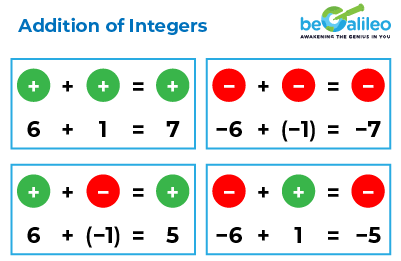## Subtraction of Integers

Subtraction of integers involves changing the sign of the second number and applying the rules of addition.

### Rules for subtracting integers:

• Subtracting a positive integer is the same as adding its negative counterpart.

Example: (+9) - (+3) = +9 + (-3) = +6

• Subtracting a negative integer is the same as adding its positive counterpart.

Example: (+6) - (-2) = +6 + (+2) = +8

Subtraction Rule Resultant Sign Example
(+) - (-) Add the absolute value of the negative integer to the positive integer + 7 - (-4) = 7 + 4 = 11
(-) - (+) Add the positive integer to the absolute value of the negative integer and give the result the sign of the negative integer. - (-7) - (+4) = -7 + (-4) = -11
(+) - (+) Subtract the second integer from the first integer. Sign of the number with the largest absolute value +7 - (+4) = 3
(-) - (-) Subtract the second integer from the first integer. Sign of the number with the largest absolute value (-7) - (-4) = -7 + 4 = -3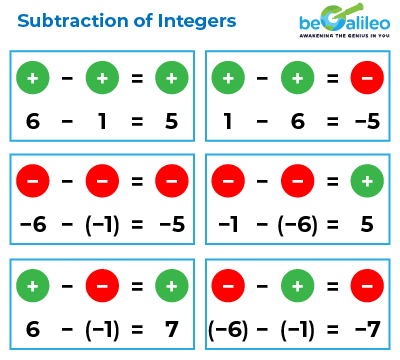## Multiplication of Integers

Multiplication of integers is finding the product of two or more integers where the value of the product depends on the integer being positive or negative. Multiplying integers is the same as multiplying whole numbers but the product has a sign.

Follow the below rules while multiplying two integers.

• If both the integers have the same sign, then the result is positive.

(-5) ✕ (-3) = 15 and 5 ✕ 3 = 15

• If the integers have different signs, then the result is negative.

(-5) ✕ 3 = -15 and 5 ✕ (-3) = -15

We can summarize the multiplication of two integers as shown below.

Multiplication Rule Resultant Sign Example
(+) × (+) Multiply the two positive integers + (+7) × (+4) = 7 × 4 = +28
(-) × (-) Multiply the two negative integers and the result is positive. + (-7) × (-4) = 7 × 4 = +28
(+) × (-) Multiply the two integers and give the result a negative sign. - (+7) × (-4) = 7 × 4 = -28
(-) × (+) Multiply the two integers and give the result a negative sign. - (-7) × (+4) = 7 × 4 = -28## Division of Integers

Division of integers is finding equal groups or dividing an integer into a specific number of groups. Dividing integers is the same as dividing whole numbers but the quotient has a sign.

The rules to divide integers are the same as in multiplication of integers.

• If both the integers have the same sign, then the result is positive.

(-24) ÷ (-8) = 3 and 24 ÷ 8 = 3

• If the integers have different signs, then the result is negative.

(-24) ÷ 8 = -3 and 24 ÷ (-8) = -3

We can summarize the division of two integers as shown below.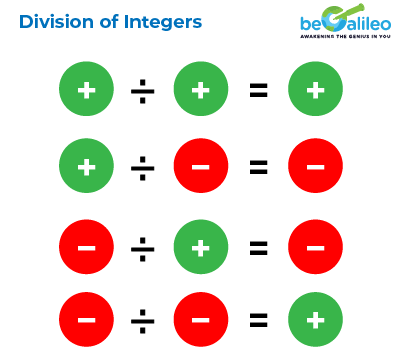Division Rule Resultant Sign Example
(+) ÷ (+) Divide the two positive integers + (+15) ÷ (+3) = 15 ÷ 3 = +5
(-) ÷ (-) Divide the two negative integers and the result is positive. + (-15) ÷ (-3) = 15 ÷ 3 = +5
(+) ÷ (-) Divide the two integers and give the result a negative sign. - (+15) ÷ (-3) = 15 ÷ 3 = -5
(-) ÷ (+) Divide the two integers and give the result a negative sign. - (-15) ÷ (+3) = 15 ÷ 3 = -5

## Properties of Integers

Integers exhibit specific properties that are essential to solve complex mathematical problems. These properties include:

• Closure Property
• Commutative Property
• Associative Property
• Distributive Property
• Multiplicative Inverse Property
• Identity Property

### Closure Property

The closure property states that the sum, difference, or product of any two integers will always result in an integer. For example, If a and b are any two integers, then a + b, a − b and a x b will also be an integer.

The closure property does not hold for division of integers.

### Commutative Property

The commutative property holds for addition and multiplication of integers, which means that changing the order of numbers does not affect the result. However, it does not hold for subtraction and division.

If a and b are any two integers, a + b = b + a and a x b = b x a

But a - b \neq b - a and a ÷ b \neq b ÷ a

### Associative Property

The associative property applies to addition and multiplication of three or more integers, stating that grouping does not affect the result. But it does not hold for subtraction and division

For example If a, b and c are any three integers,

• a + (b + c) = (a + b) + c
• a x (b x c) = (a x b) x c

### Distributive Property

The distributive property states that when you multiply an integer by the sum or difference of two other integers, it's the same as if you had multiplied the integer by each of those integers individually and then added or subtracted the results.

For example, If a, b and c are any three integers, then,

• a x (b + c) = (a x b) + (a x c)
• a x (b - c) = (a x b) - (a x c)

The additive inverse property states that the addition of an integer and its negative will result in zero.

In other words, for any integer a, there exists an integer -a such that a + (-a) = 0.

### Multiplicative Inverse Property

The multiplicative inverse property states that multiplying an integer by its reciprocal, always results in 1.

For any integer a, there exists a multiplicative inverse \frac{1}{a} such that a x \frac{1}{a} = 1.

### Identity Property

The identity property states that adding or subtracting zero from an integer leaves the integer unchanged.

For example, If a is an integer, then,

a + 0 = a and a - 0 = a

Similarly, When an integer is multiplied or divided by 1, it will result in the integer itself.

For example, If a is an integer, then,

a x 1 = a and a ÷ 1 = a

## Solved Examples

### Q1. Find the sum of (-3) and 7.

1. a) 4
2. b)-4
3. c) 10
4. d)-10

Solution: The correct answer is a) 4
The sum of two integers with different signs is found by subtracting their absolute values and giving the result the sign of the integer with the greater absolute value.
(-3) + 7 = 7 - 3 = 4

### Q2. Evaluate the expression (-2) × (-5).

1. a)-10
2. b) 10
3. c) -7
4. d) 3

Solution: The correct answer is: b) 10
The product of two integers with the same sign is always positive.
(-2) × (-5) = 2 × 5 = 10

### Q3. Calculate (-14) ÷ 2.

1. a) 7
2. b) 16
3. c) 28
4. d) -7

Solution: The correct answer is: d) -7
The quotient of two integers with different signs is always negative.
(-14) ÷ 2 = -7

### Q4. Simplify the expression: -4 × (-5 + 3).

1. a) 23
2. b) 32
3. c) 8
4. d) 0

Solution: The correct answer is: c) 8
Apply the distributive property first and then perform the operation.
-4 × (-5 + 3) = -4 × -2 = 8

### Q5. Find the additive inverse of 11.

1. a) -11
2. b) 22
3. c) 11
4. d) None of these

Solution: The correct answer is: a) -11
The additive inverse of an integer 'a' is '-a.'
Additive inverse of 11 is -11

## FAQ on Integers

The main types of integers are positive integers, negative integers, and zero. Positive Integers: Integers greater than zero, denoted as (+1, +2, +3, ...). Negative Integers: Integers less than zero, denoted as (-1, -2, -3, ...). Zero: The neutral integer, denoted as 0.

The set of integers is represented by the symbol "ℤ".

The commutative property holds for addition and multiplication of integers, meaning that changing the order of numbers does not affect.

To compare integers on the number line, move to the right for positive integers and to the left for negative integers. The greater the absolute value of an integer, the farther it is from zero.

The multiplicative identity property of integers states that any integer multiplied by one equals the original integer. It means that multiplying an integer by one does not change its value.

The additive inverse property states that the addition of an integer and its negative will result in zero. For example, the additive inverse of 8 is -8 because 8 + (-8) = 0.

In finance, positive integers are used to represent credits or gains, while negative integers represent debts or losses. For example, if you deposit $100 into your bank account, it would be represented as +100, and if you owe$50 on a credit card, it would be represented as -50.

No, fractions and decimals are not considered integers. Integers only consist of whole numbers and their negatives.

To understand changes in temperature, you can use positive and negative integers. For example, if the temperature increased by 5 degrees Celsius, you can represent it as +5°C. Conversely, if the temperature decreased by 3 degrees Celsius, you can represent it as -3°C.

Consecutive integers are integers that follow one another in order, with a difference of 1 between each consecutive pair. For example, 3, 4, and 5 are consecutive integers.

When adding a positive integer and a negative integer, find the difference between their absolute values and give the result the sign of the integer with the greater absolute value. If the positive integer has a greater absolute value, the sum is positive; if the negative integer has a greater absolute value, the sum is negative.

To subtract a positive integer from a negative integer, add the absolute values of both integers and give the result the sign of the integer with the greater absolute value. If the negative integer has a greater absolute value, the difference is negative; if the positive integer has a greater absolute value, the difference is positive.

Yes, whole numbers are a subset of integers. Whole numbers consist of all positive integers (including zero), but they do not include negative integers. In other words, all whole numbers are integers, but not all integers are whole numbers. For example, 0, 1, 2, 3, ... are whole numbers and integers, while -1, -2, -3, ... are integers but not whole numbers.

#